Latest Banking jobs   »   IBPS quant

# Quantitative Aptitude Quiz For IBPS RRB PO/Clerk Mains 2023-07th-September

Directions (1-5):-Each of the following question is followed by two quantities I, and II. You have to determine the value of the quantities using the information provided and compare the quantities to answer as per the instruction set provided below.
(a) Quantity I>Quantity II
(b) Quantity I≥Quantity II
(c) Quantity I<Quantity II
(d) Quantity I≤Quantity II
(e) Quantity I=Quantity II or no relation

Q1. What is the number?
Quantity I: 40% of number is 12.
Quantity II: 50% of 20 is number.

Q2. Find the time taken to cover 30 km.
Quantity I: the speed of bike is 20 kmph.
Quantity II: a car covers 20 km in 30 minutes.

Q3. Find the present population of town.
Quantity I: the population increases every year by 10% and last year it was 10000.
Quantity II: male population is 30% more than female population which is 5000.(population= male+ female)

Q4. Quantity I: marks obtained in Maths is 90 which is 10% less than marks obtained in Science. What is average marks in both subjects?
Quantity II: 95

Q5. Quantity I: Prem saves Rs. 5000 per month from annual salary of Rs. 180000. Find expenditure per month.
Quantity II: Rahul spends at least 60% of his income and saves Rs. 4000 per month. What can be his monthly salary?
Directions (6-10): In the given questions, two quantities are given, one as ‘Quantity I’ and another as ‘Quantity II’. You have to determine relationship between two quantities and choose the appropriate option:

Q6. Ratio of age of Anurag and Bhumi is 6 : 7 and Dharmendra : Ekta is 9 : 5. Ratio of average age of Anurag, Bhumi, Dharmendra& Ekta to age of Chiru is 9 : 10
Quantity I– If ratio of age of Bhumi and Dharmendra is 7 : 9 and age of Chiru is 6 years less than age of Dharmendra, then age of Chiru.
Quantity II – If ratio of age of Bhumi and Dharmendra is 7 : 9 and age of Chiru is 6 years less than age of Dharmendra, Average age of Anurag& Dharmendra.
(a) Quantity I > Quantity II
(b) Quantity I < Quantity II
(c) Quantity I ≥ Quantity II
(d) Quantity I ≤ Quantity II
(e) Quantity I = Quantity II or no relation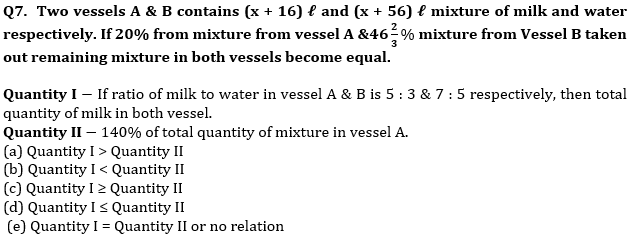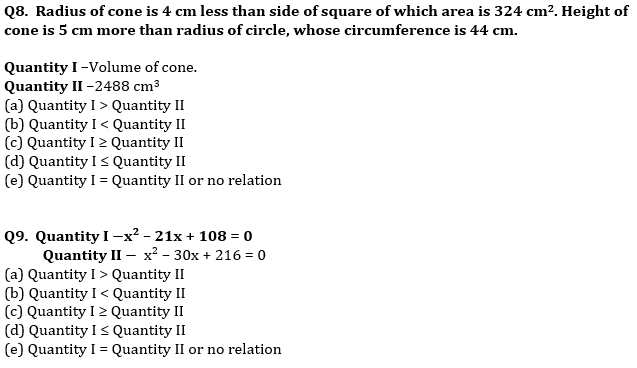Q10. 240 meters & 210 meters long trains running in opposite direction cross each other in 6 sec. Ratio between speed of longer train and smaller train is 7 : 8 and faster train cross a platform in 9 sec.
Quantity I – Time taken (in sec) by slower train to cross a bridge, which is 60 meters longer than platform.
Quantity II –16 sec
(a) Quantity I > Quantity II
(b) Quantity I < Quantity II
(c) Quantity I ≥ Quantity II
(d) Quantity I ≤ Quantity II
(e) Quantity I = Quantity II or no relation

Solutions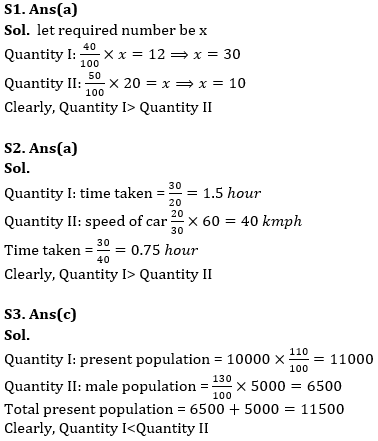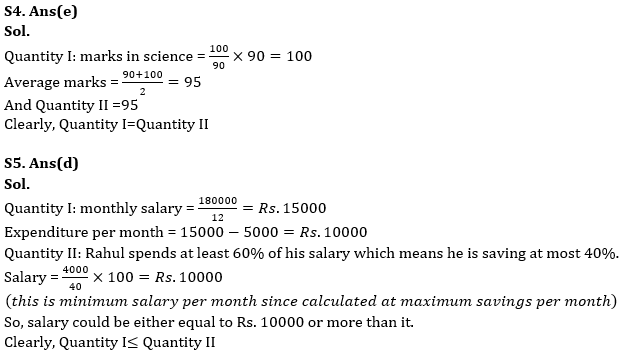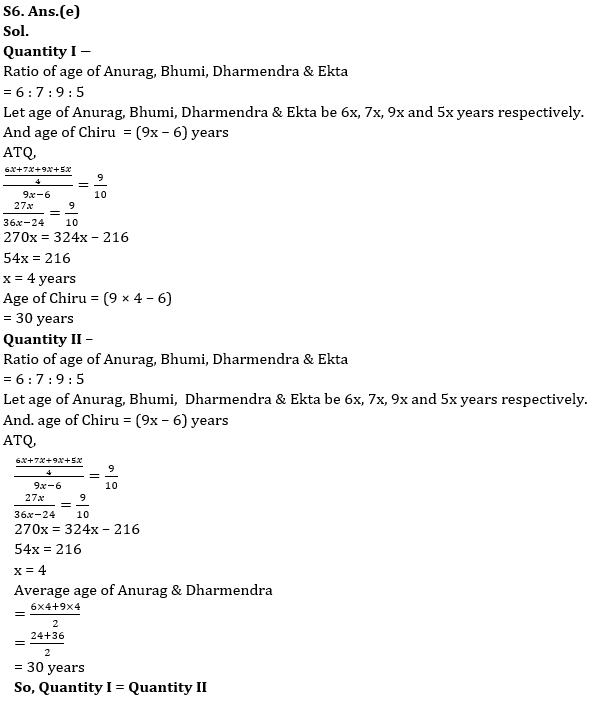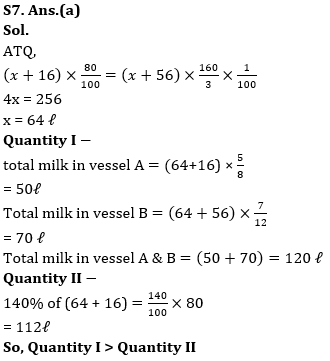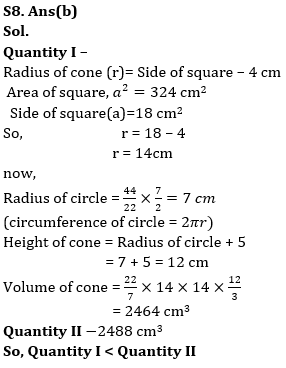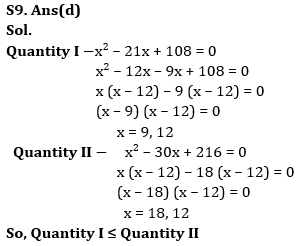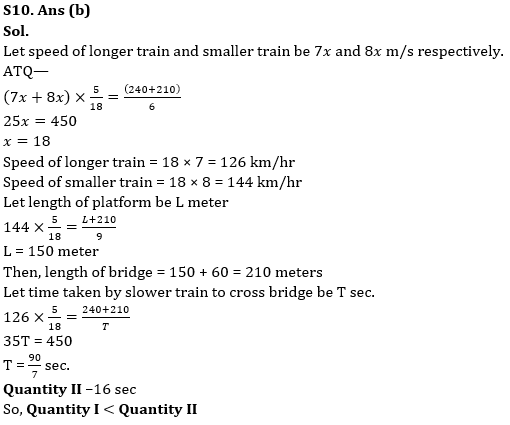## FAQs

### When will the IBPS RRB PO mains exam be conducted?

IBPS RRB PO mains exam will be conducted on 10 September 2023

#### Congratulations!Union Budget 2023-24: Free PDF